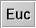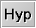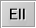# Geometriesbacklinks... Composition of Transformations Dynamic Geometry Polygons Table of Contents The Main Menu Transformation Groups Views What Is New in Cinderella.2 What Is New in Cinderella.2J English 日本語

## Geometries

It is one of Cinderella's main features that it supports different kinds of geometries. If you are not accustomed to the idea that there are "different kinds of geometry," this may sound confusing. You should read the section Theoretical Background to get a feeling for the underlying ideas and how they are implemented in Cinderella. Users who already have some basic knowledge about Euclidean and non-Euclidean geometries might be satisfied reading this reference part only.

Another warning message: Do not confuse geometries with views; they both come in similar flavors, but the first defines the behavior of elements, while the second describes the presentation of elements.

### Types of Geometry

In each main window of Cinderella you will find three buttons for choosing the type of geometry. In the present version, Cinderella provides three different kinds of geometry: Euclidean geometry, hyperbolic geometry, and elliptic geometry. You can switch among these three geometries by pressing the buttonfor Euclidean geometry,for hyperbolic geometry, orfor elliptic geometry.

The choice of a new geometry does not affect the behavior of the elements you have already constructed. However, every newly added element is interpreted with respect to the new geometry. You can think of each element as having an entry that tells it to which geometry it belongs. The basic notions affected by the choice of the geometry are the measurements of distances and angles.

However, also other constructions are influenced by this choice. For instance, the angle bisector is defined to be a line whose angles to two other lines are equal. If the measurement of angles has changed, then the definition of angle bisector has to change, too. Similar things happen to "parallels" and "perpendicular lines." The definition of a circle is also influenced by the geometry. A circle is the set of all points that have the same given distance to the center. If the notion of "distance" is changed, the concept of "circle" changes as well.

Other operations are not affected by the choice of the geometry at all: the line joining two points will always be the same no matter in which of the above geometries you are.

The following list collects all constructions that are influenced by the choice of the geometry. Observe that the position, as well as the number, of elements that are constructed can change.

• Distance: The notion of distance depends on the geometry. It can even happen that in hyperbolic geometry distances of real points become complex numbers, for instance when the line joining both points lies completely outside the horizon.
• Angle: The notion of angle depends on the geometry. As in the case of distances, angles can also become complex numbers.
• Circle: The exact notion of circularity depends on the definition of "distance," which changes in each geometry. This influences all construction modes for circles. In the Euclidean view, hyperbolic or elliptic circles can look like arbitrary conics. The picture is clarified in the other views. In the hyperbolic view (Poincaré disk), hyperbolic circles really look like circles. In the spherical view, elliptic circles look like circles on the surface of a ball.
• Mirror: The notion of reflection depends on distances and angles and thus it depends on the geometry. All kinds of mirrors are influenced this way.
• Angle bisector: In all three geometries, you have two angle bisectors for a line. However, the exact position depends on the choice of the geometry. In hyperbolic geometry, angle bisectors of real lines can become complex.
• Midpoint: The midpoint of two points depends on the definition of "distance." In Euclidean geometry, there is exactly one such midpoint. In hyperbolic and elliptic geometry there are two such midpoints (points of equal distance to the defining points). Caution: If you are in the "hyperbolic view," only one of these points will be visible, since the other one lies outside the horizon.
• Line with fixed angle: This construction is influenced by the choice of the geometry because angles are involved.
• Perpendicular: The notion of a perpendicular depends on the notion of "angle" and is influenced by the choice of the geometry.
• Parallel: In Cinderella parallels of a line L are defined as lines that have an angle of zero to L. In Euclidean geometry there is a unique parallel to L through a point. However, in hyperbolic and elliptic geometry there are two such parallels in general. The parallels in elliptic geometry will usually have complex coordinates, so that you will see them only in the "construction text" view.

In the present version of Cinderella some operations are not supported in all geometries. These operations are Circle by Three Points, Area, and Center, where the Euclidean result is always calculated.

### Views and Geometries

Although every geometry is usable together with every view, a few combinations are a bit more common than others. Here is a short list of what these common choices represent.

Euclidean view in Euclidean geometry:
This may be the most common choice. The geometric elements behave like "usual elements" in a "usual plane."

Spherical view in Euclidean geometry:
This choice gives you control over the behavior "at infinity" of the Euclidean plane. The spherical view represents a double cover of the Euclidean plane. Each line is mapped to a great circle and each point is mapped to an antipodal pair of points. The boundary of the nonrotated view corresponds to the "line at infinity" of the Euclidean plane.

Euclidean view in hyperbolic geometry:
What you see here is the "Beltrami-Klein" model of hyperbolic geometry. In this model hyperbolic lines are really straight. Measurement is done according to the definitions of the Cayley-Klein geometry. In the Euclidean view the horizon of hyperbolic geometry is shown as a thin circle.

Hyperbolic view in hyperbolic geometry:
This is what is known as the Poincaré disk. Hyperbolic lines are represented by circular arcs that cross the boundary of the disk at right angles. The Poincaré disk distorts the usual plane in a way that hyperbolic angles between lines correspond to "Euclidean" angles between the corresponding circular arcs. In mathematical terms, the Poincaré disk is a conformal representation of the hyperbolic plane. In this picture hyperbolic circles really look circular.

The whole disk represents only a part of the full plane of the corresponding Cayley-Klein geometry. The part that is shown corresponds to the region inside the circle shown in the Euclidean view. The measurement of distances is such that the distance from any interior point to any point on the boundary is equal to infinity.

Spherical view in elliptic geometry:
The spherical view is the natural view for elliptic geometry. The angle between two lines corresponds to the spherical angles of the corresponding great circles. Measurement of distances corresponds to geodesic measurement of distances on the surface of a ball. Elliptic circles correspond to circles on the surface of the ball.

However, one has to be a bit careful. Elliptic geometry is not equivalent to spherical geometry (geometry on the ball). This comes from the fact that in elliptic geometry antipodal points of the ball are identified with each other.